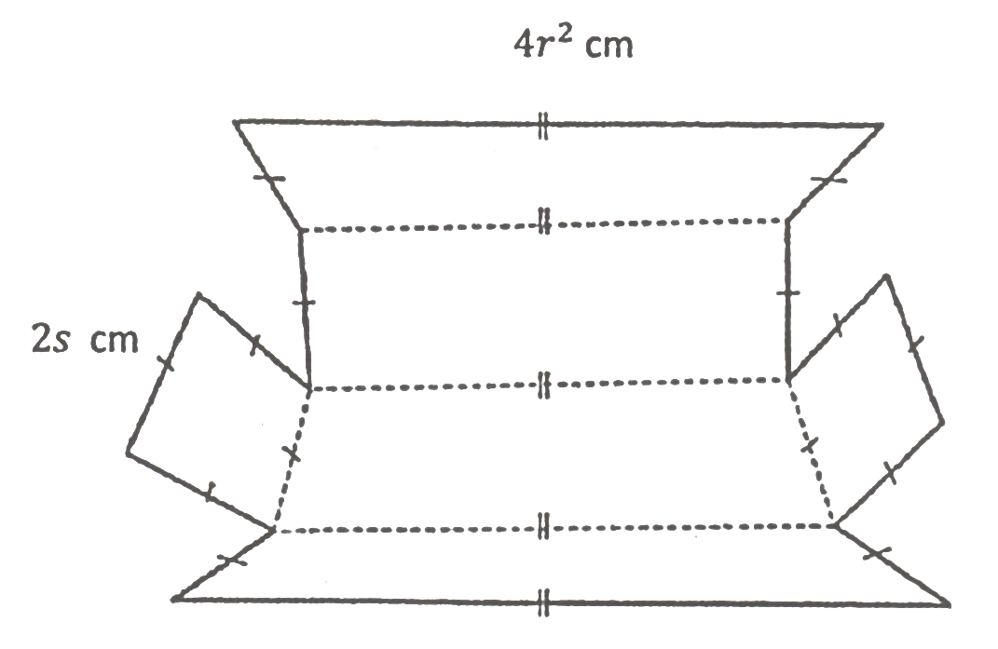# SPM Mathematics Trial 2021 (Kelantan), Paper 2 (Question 5 & 6)

Question 5:
(a) Determine whether the following arguments are valid or invalid. For valid arguments, determine whether it is sound or not sound: (2 marks)

 Premise 1: All prime numbers are odd numbers Premise 2: 3 is the prime numberConclusion: 3 is an odd number
(b) From an implication ” p if and only if q ” for the implication below : (2 marks)

 If a is an odd number, then a cannot be divided exactly by 2

Solution:
(a)
The arguments are valid but not sound.

(b)
Implication: a is an odd number, if and only if a cannot be divided exactly by 2.

Question 6 (4 marks):
Diagram 3 shows an unfolded cuboid box.Diagram 3

Express the total surface area of the cuboid in terms of r and s, then calculate the volume, in cm3, of the box if r = 4 cm and s = 6 cm.

Solution:
Total surface area of the cuboid
= 4 (4 r2×2 s) + 2 (2 s × 2 s)
= 4 (8 r2 s) + 2 (4s2)
= 32 r2 s + 8 s2

Volume of the box
= Length × Width × Height
= 4r2 × 2s × 2s
= 4(4)2 × 2(6) × 2(6)
= 4(16) × 12 × 12
= 9 216 cm3